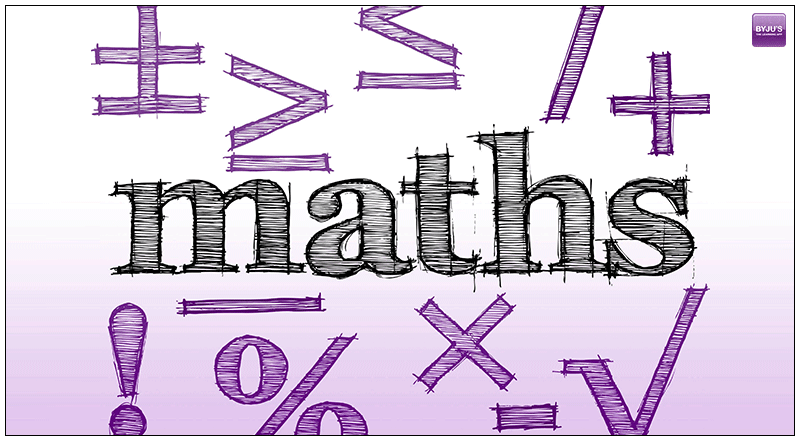# GMAT Maths – Mixtures & AlligationMixture problems can also be called as word problems where quantities or items of diverse significances are mixed together. At times, different liquids can be mixed together to change the concentration of the mixture. Or, occasionally, different costs can be mixed together to get some different value.

The problems of mixture are an excellent candidate for solving a problem through a system of equations. These mixture problems can be easily solved if only you could derive the equations out of the problem statements.

The most recommended method by all math experts, of solving a mixture problem is through preparing a table. By drawing a table, it gives you a broader idea of how to use a number one at a time in playing of getting jumbled up in all the information available.

Let’s work through some of the mixture problems now and learn the tactics of to treating them.

Problem 1. Jack has 30 grains of a 30% of a salt solution. What amount of salt should be added to make it a 35% solution?

Solution:

1. First, create a table for the salt solution.
 Original amount Amount added result Concentration of salt amount

1. As the information is given in the question, fill out the table.

Here,

Let the salt added = 100%, which can be taken as 1 in decimal form.

Now change all the percent value into decimals form

Let x = amount of salt that has been added. So, the result would become 30 + x.

 Original amount Amount added result Concentration of salt 0.3 1 0.35 amount 30 x 30+x
1. Multiply down the each column
 Original amount Amount added result Concentration of salt 0.3 1 0.35 amount 30 x 30+x multiply 0.3× 30 1 ×x 0.35 ×(30+x)
1. Original Amount + Amount added = result

0.3× 30 + 1 ×x = 0.35 ×(30+x)

9 + x = 10.5 + 0.35x

0.65x = 1.5

x = 2.307 grains

So, the amount of salt to be added is 2.307 grains

We’ll be glad to help you in your GMAT preparation journey. You can ask for any assistance related to GMAT and MBA from us by calling us at +918884544444. You can write to us at gmat@byjus.com.